# Introduction to canopy RT modelling¶

## J Gómez-Dans (UCL & NCEO)¶

• In the previous Session we looked at RT modelling of leaves
• Now we will consider a full canopy
• We will just the basics, as the aim is that you can start using well-established RT models
• Obviously, lots more to cover!
• We will mostly consider a turbid medium canopy
• That is a random volume of leaves and air
• In the optical domain, the size of the objects is $>>\lambda$### Define the canopy¶

• Vertical leaf area density function $u_{L}(z)\,\left(m^{2}m^{-3}\right)$,
• Vertical leaf number density function (e.g. the number of particles per unit volume), $N_{v}(z)\,\left(N\,particles\, m^{-3}\right)$
$$LAI = \int_{z=0}^{z=H} u_{L}(z)dz$$
• Distribution of the leaf normal angles, $g_{L}(z, \vec{\Omega}_{L})$ (dimensionless),
• Leaf size distribution, defined as area density to leaf number density and thickness.#### Some examples of leaf angle distribution functions¶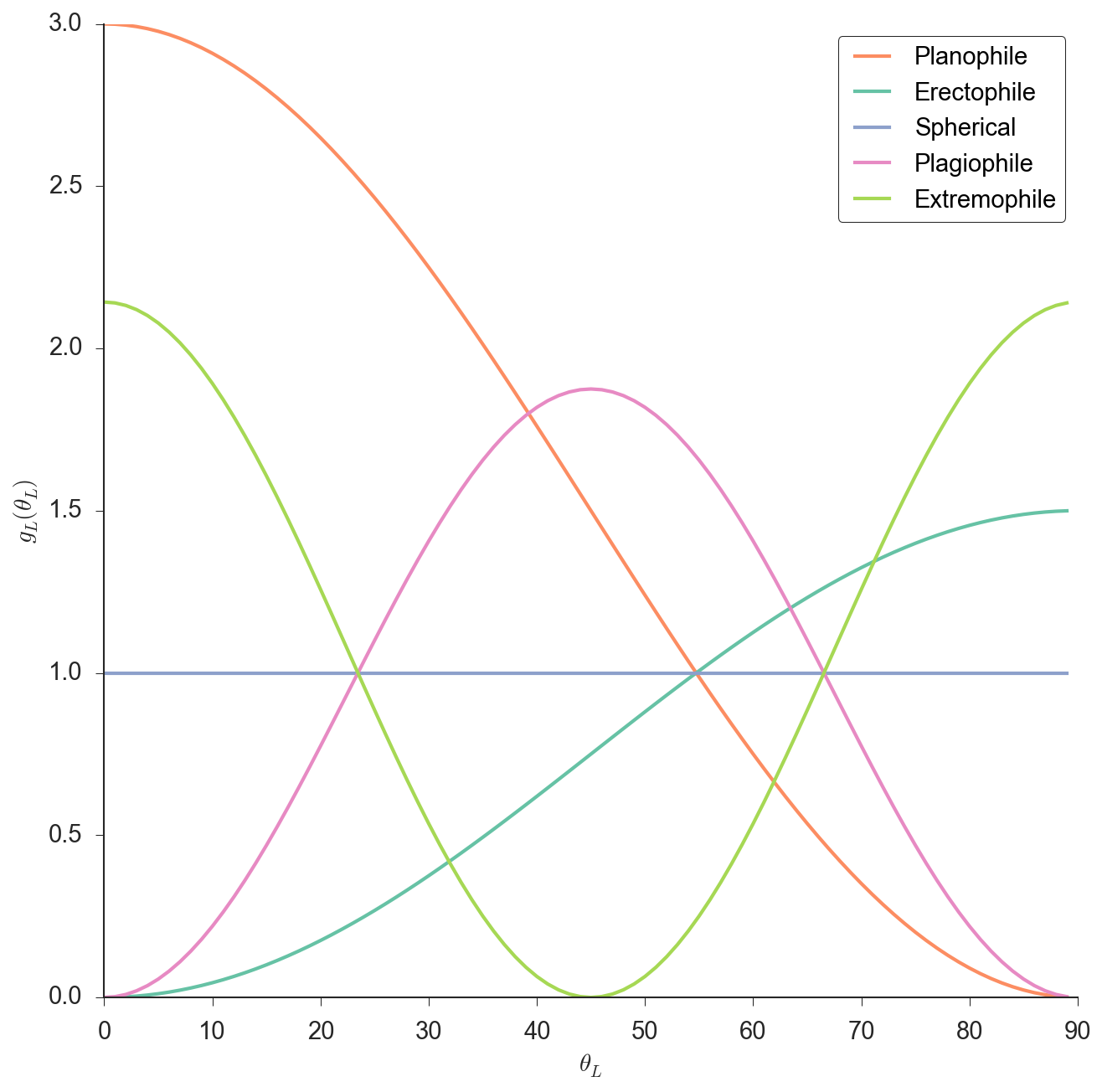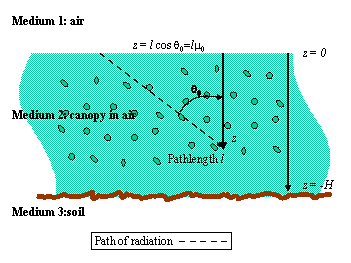The RTE describes the change of incident radiance intensity at a specific height and direction $I(z,\vec{\Omega})$.

$$\mu\cdot\frac{\partial I(z,\vec{\Omega})}{\partial z} = \overbrace{-\kappa_{e}\cdot I(z,\vec{\Omega})}^{\textsf{Attenuation}} + \underbrace{J_{s}(z,\vec{\Omega})}_{\textsf{Volume scattering}},$$

### Attenuation¶

• Attenuation is governed by Beer's Law $$I(z)=I(0)\exp(-\kappa_{e}\cdot z)=I(0)\exp(-\kappa_e\cdot LAI)$$
• $\kappa_{e}$ is the product of the medium's particle density and the extinction x-section
• Also split into radiation absorbed and scattered away in other directions.
• Remember that $LAI = \int_{z=0}^{z=-H}u_{L}(z)dz$
• Q What value of LAI is needed to intercept 99% of the radiation if $\kappa=1$?
• What if your particles (leaves) are oriented?
• We need to project the leaf area density across the beam direction $\vec{\Omega}$
• We project the leaf angle distribution function $u_{L}(z)$ using into $\vec{\Omega}$ by multiplying by
$$G(\vec{\Omega})=\int_{2\pi} g_{L}(\vec{\Omega}')\left| \vec{\Omega}\cdot\vec{\Omega}'\right| d\vec{\Omega}'.$$

$$G(\vec{\Omega})=\int_{2\pi} g_{L}(\vec{\Omega}')\left| \vec{\Omega}\cdot\vec{\Omega}'\right| d\vec{\Omega}'.$$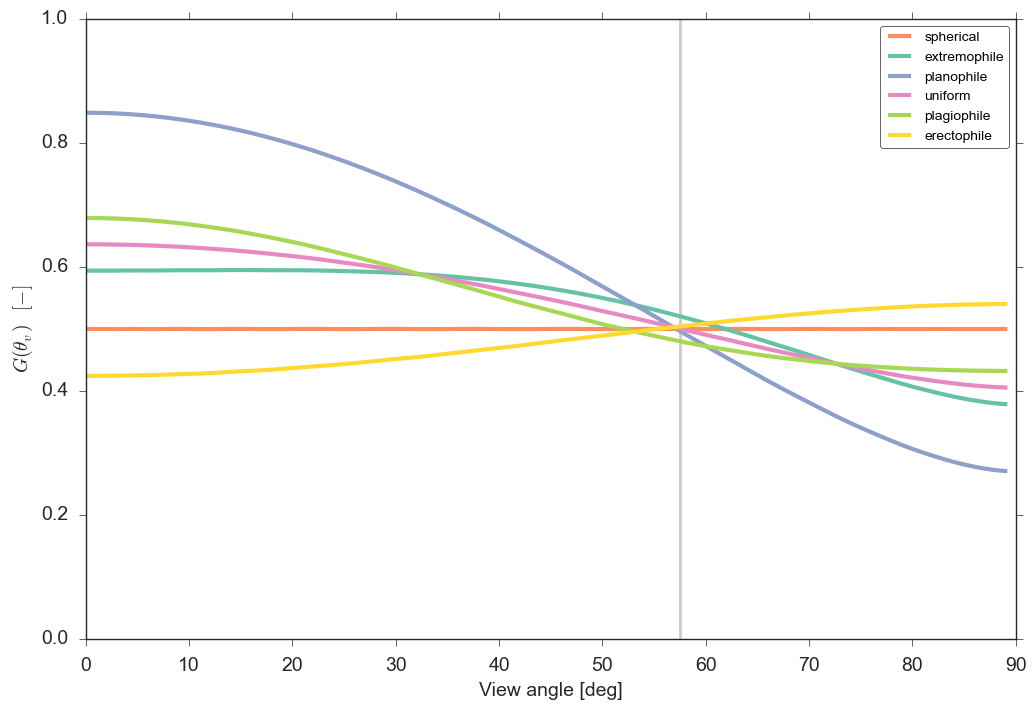• From the previous slide, we have that
$$I(z)=I(0)\exp\left[-u_{L}\cdot G\left(\vec{\Omega}\right)\cdot \frac{z}{\mu}\right],$$

where $\kappa_{e}=G(\vec{\Omega})/\mu$.

So...

• Attenuation is a function of
• Leaf area
• leaf angle distribution
• direction of propagation
• What happens to $\kappa_e$ when...
• Sun is overhead, vertical leaves?
• Leaves are horizontal?
• Zenith angle is low?
• Zenith angle is high?
• At around $\sim 1 rad$ angle?

### Volume scattering¶

$$J_{s}(z,\vec{\Omega}) = \int_{4\pi} P(z,\vec{\Omega}'\rightarrow\vec{\Omega})\cdot I(\vec{\Omega}',\vec{\Omega})d\vec{\Omega}' ,$$
• Indicates scattered incoming radiation from all directions into the viewing direction $\vec{\Omega}$.
• $P(\cdot)$ is the phase function
• In the optical domain, we tend to specify $P(\cdot)$ as a function of leaf area density and an area scattering function $\Gamma(\cdot)$.
\begin{align} \Gamma\left(\vec{\Omega}'\rightarrow\vec{\Omega}\right) &= \frac{1}{4\pi}\int_{2\pi+}\rho_{L}(\vec{\Omega}', \vec{\Omega})g_{L}(\vec{\Omega_{L}})\left|\vec{\Omega}\cdot\vec{\Omega}_{L} \right|\left|\vec{\Omega}'\cdot\vec{\Omega}_{L} \right|d\vec{\Omega}' \\\\ &+ \frac{1}{4\pi}\int_{2\pi-}\tau_{L}(\vec{\Omega}', \vec{\Omega})g_{L}(\vec{\Omega_{L}})\left|\vec{\Omega}\cdot\vec{\Omega}_{L} \right|\left|\vec{\Omega}'\cdot\vec{\Omega}_{L} \right|d\vec{\Omega}' \\ \end{align}
• $\Gamma$ is a double projection of the leaf angle distribution, modulated by the directional reflectance (upper hemisphere) and transmittance (lower hemisphere)
• This is quite similar to $G$
• Typically, we assume leaves to be bi-Lambertian, so simplify.... $$\Gamma\left(\vec{\Omega}'\rightarrow\vec{\Omega}\right) = \rho_{L}\cdot\Gamma^{\uparrow}(\vec{\Omega}, \vec{\Omega}') + \tau_{L}\cdot\Gamma^{\downarrow}(\vec{\Omega}, \vec{\Omega}')$$

• Also, if we assume $\rho\sim\tau$ (or a linear function), $\Gamma$ is a weighting of the upper and lower double projections of the leaf angle distribution modulated by the spectral properties of the single scattering albedo.

### Solving the RTE¶

• Expressions for attenuation and scattering $\Rightarrow$ can solve the RTE.
• Need a bottom boundary (=soil)
• Assume only the first interaction (only one interaction with canopy or soil)
• I will skip over the algebra to give an expression for the directional reflectance factor:
\begin{align} \rho(\vec{\Omega}_{s}, \vec{\Omega}_{o}) =& \exp\left\lbrace -L\cdot\left[ \frac{G(\vec{\Omega}_{s})\mu_{o} + G(\vec{\Omega}_{o})\mu_{s}} {\mu_s\mu_o} \right]\right\rbrace \cdot \rho_{soil}(\vec{\Omega}_{s}, \vec{\Omega}_{o}) \\\\ &+ \frac{\Gamma\left(\vec{\Omega}'\rightarrow\vec{\Omega}\right)}{G(\vec{\Omega}_{s})\mu_{o} + G(\vec{\Omega}_{o})\mu_{s}}\cdot\left\lbrace 1 - \exp\left[ -L\cdot\left( \frac{G(\vec{\Omega}_{s})\mu_{o} + G(\vec{\Omega}_{o})\mu_{s}} {\mu_{s}\mu_{o}} \right)\right]\right\rbrace\\\\ \end{align}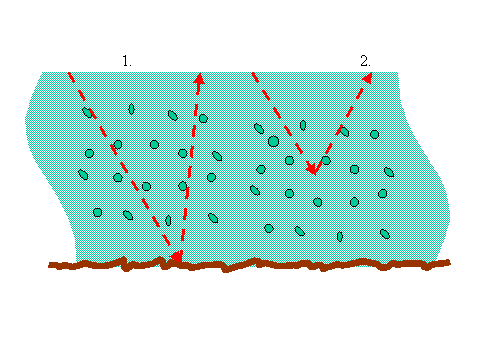#### 2 terms!¶

1. $\exp\left\lbrace -L\cdot\left[ \frac{G(\vec{\Omega}_{s})\mu_{o} + G(\vec{\Omega}_{o})\mu_{s}} {\mu_s\mu_o} \right]\right\rbrace \cdot \rho_{soil}(\vec{\Omega}_{s}, \vec{\Omega}_{o})$
• Radiation travelling through the canopy $\rightarrow$ hitting the soil $\rightarrow$ traversing the canopy upwards
• Double attenuation is given by Beer's Law, and controlled by LAI and leaf angle distribution
2. $\frac{\Gamma\left(\vec{\Omega}'\rightarrow\vec{\Omega}\right)}{G(\vec{\Omega}_{s})\mu_{o} + G(\vec{\Omega}_{o})\mu_{s}}\cdot\left\lbrace 1 - \exp\left[ -L\cdot\left( \frac{G(\vec{\Omega}_{s})\mu_{o} + G(\vec{\Omega}_{o})\mu_{s}} {\mu_{s}\mu_{o}} \right)\right]\right\rbrace$
• Volumetric scattering of the canopy
• Controlled by area scattering phase fanction $\rightarrow$ control by single scattering albedo
• Inverse dependency in $G$ and view-illumination angles
• Dependence on LAI too
• When can we ignore the contribution of the soil?

### (Yet) more simplifying assumptions...¶

• Assume a spherical leaf angle distribution function & bi-Lambertian leaves
• What does this mean?
• If reflectance is assumed to be linearly related to transmittance $k = 1 + \tau_{L}/\rho_{L}$
$$\Gamma\left(\vec{\Omega}'\rightarrow\vec{\Omega}\right) = \frac{\rho_{L}k}{3\pi}\left[\sin\gamma + \left(\frac{\pi}{k}-\gamma\right)\cos\gamma\right]$$
• $\cos\gamma=\left|\vec{\Omega}'\cdot\vec{\Omega}\right|$
$$\Gamma\left(\vec{\Omega}'\rightarrow\vec{\Omega}\right) = \frac{\rho_{L}k}{3\pi}\left[\sin\gamma + \left(\frac{\pi}{k}-\gamma\right)\cos\gamma\right]$$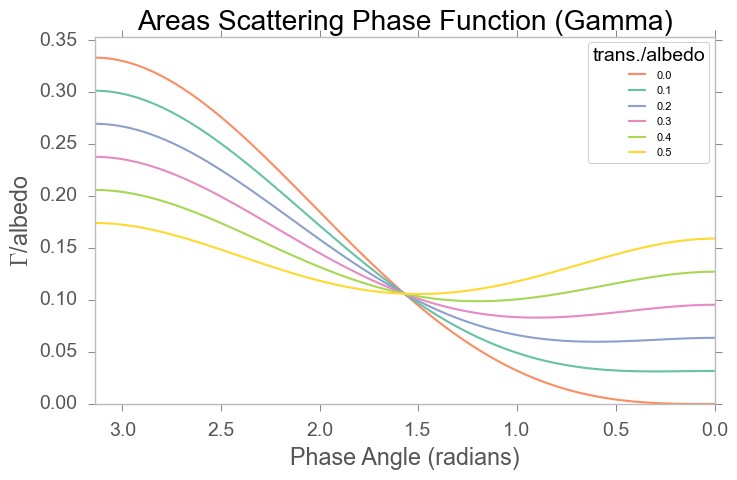### Recap¶

• For first order solution, $\rho(\Omega, \Omega')$...
• Refl factor combination of two tersm: uncollided direct & collided volume term
• double attenuated soil return (dependent on leaf angle distribution, LAI, view/illum angles)
• volume scattering: as above, but also dependent on leaf optical properties
• Tends to be larger for larger phase angles
• Remember all the assumptions we made!!!
• In the NIR, $\omega$ is quite high, need multiple scattering terms!

### Multiple scattering¶

• Range of approximate solutions available
• Successive orders of scattering (SOSA)
• 2 & 4 stream approaches etc. etc.
• Monte Carlo ray tracing (MCRT)
• Recent advances using concept of recollision probability, $p$

### LAI = 1¶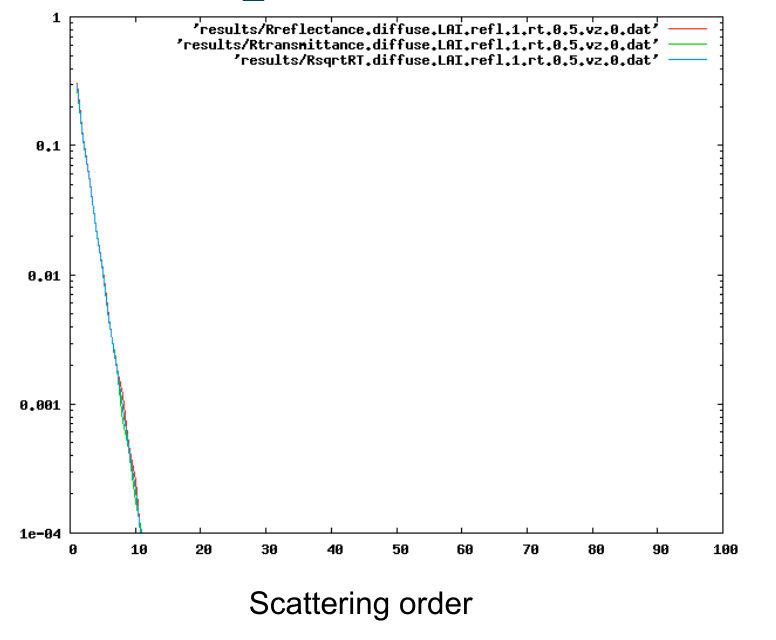### LAI = 5¶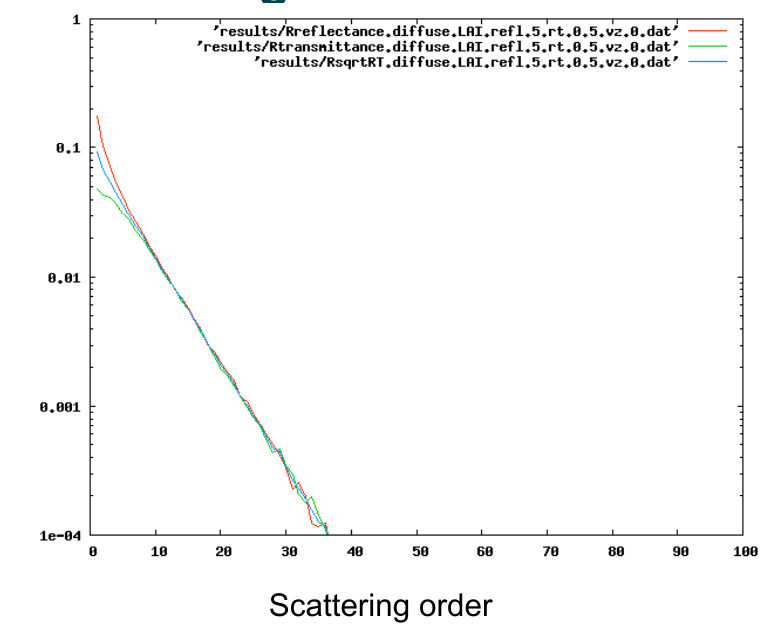### LAI = 8¶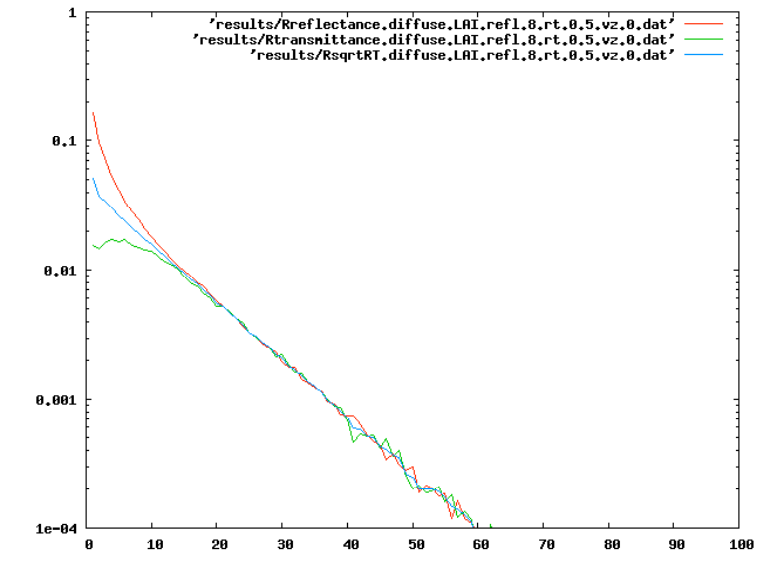#### Recollision probability¶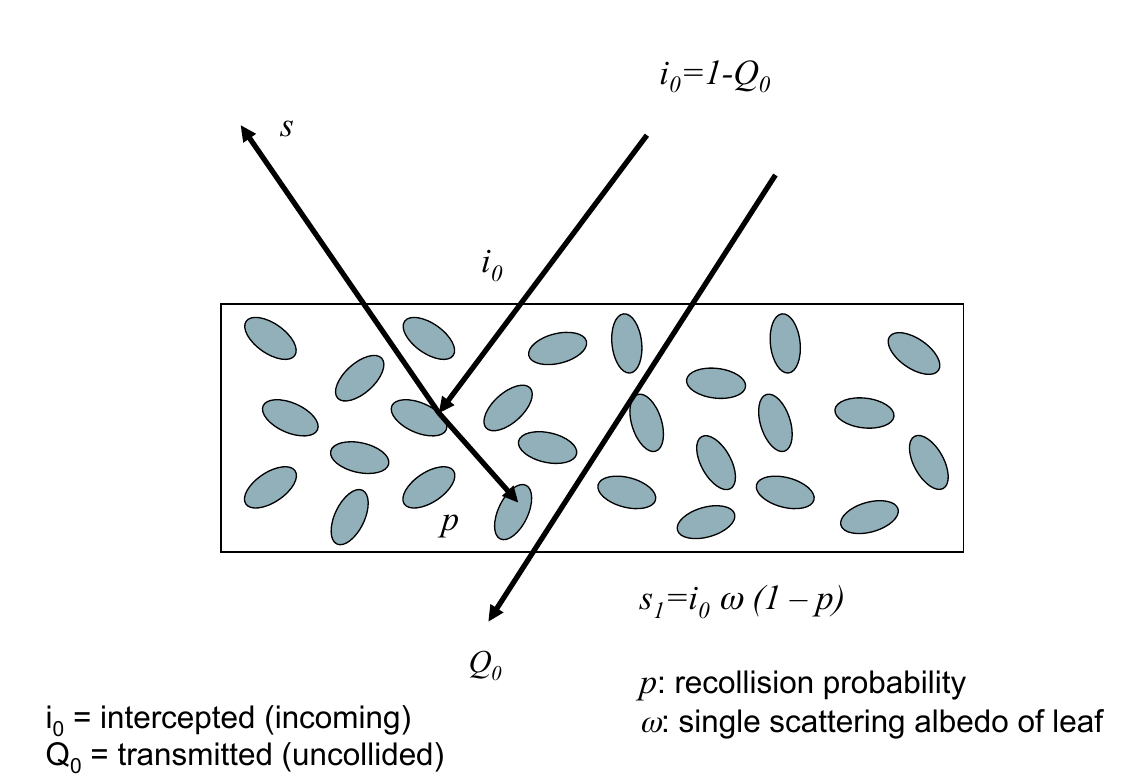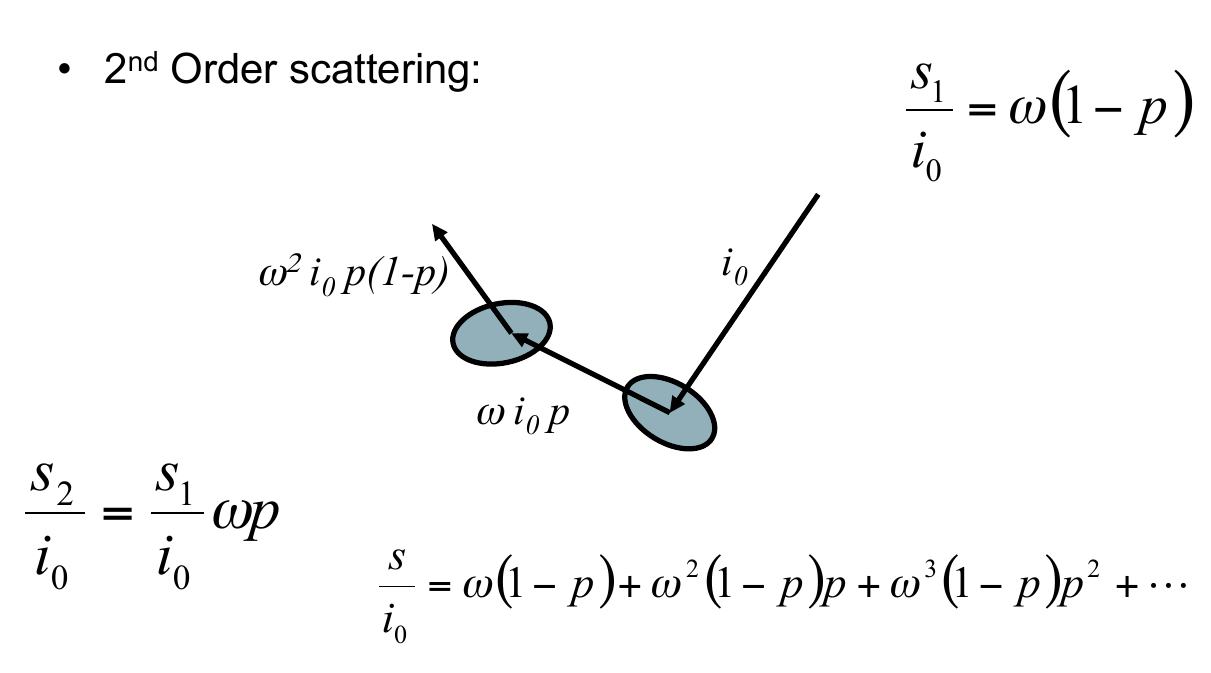\begin{align} \frac{s}{i_0}&=\omega(1-p) + \omega^2(1-p)p + \omega^2(1-p)p^2 + \dots\\\\ &=\omega(1-p)\left[ 1 + \omega p + \omega^2p^2 + \dots\right]\\\\ &=\frac{\omega(1-p)}{1-p\omega} \end{align}
• $p$ is en eigenvalue of the RT equation $\Rightarrow$ only depends on structure
• We can use this form to describe reflectance if black soil (or dense canopy)
• From Smolander & Sternberg (2005), $$p = 0.88 \left[ 1 - \exp(- 0.7 LAI^{0.75}) \right]$$
• Assuming spherical leaf angle distribution

### The hotspot effect¶

• The term $$\exp\left[-L\frac{G(\vec{\Omega}_s)\mu_o + G(\vec{\Omega}_o)\mu_s}{\mu_s\cdot\mu_o}\right]$$ is usually called the joint gap probability, and is the probability of a photon traversing the canopy downwards and then upwards without a collision.
• We have assumed these two probabilities are independent, which holds in general...
• but what happens if we consider the retroreflection (=backscatter) direction?
• Then, the downward and upward probabilities need to be identical!
• We need a correction factor for the hotspot direction!
• The increased gap probability results in an enhancement of the reflectance factor (the hotspot)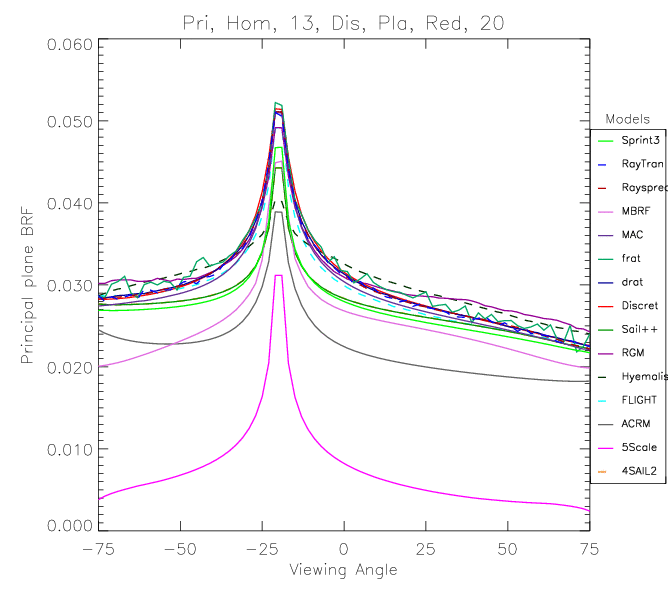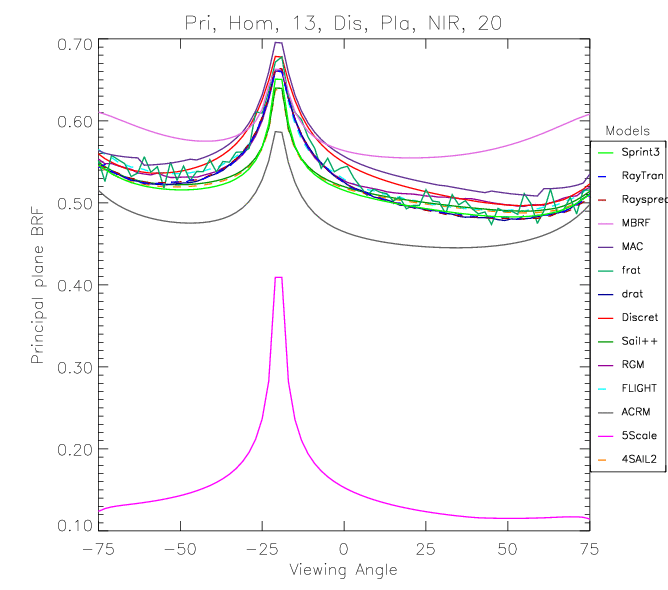### Discontinous canopies¶

• We have looked at a turbid medium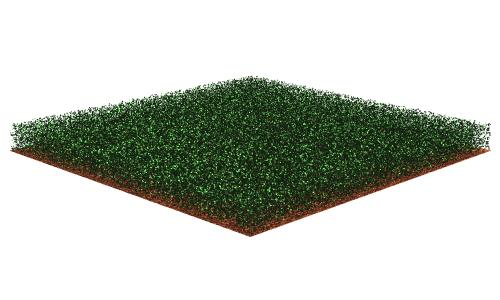• This might be acceptable for a grass canopy such as cereals
• But clearly not right for a savanna!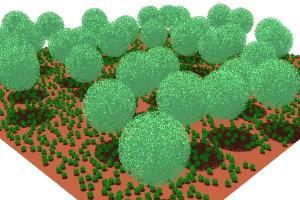• Clumping of the canopoy can be encoded as a modulation on LAI, $C$
• So the gap fraction becomes $$\exp\left(-L\cdot C\frac{G(\Omega)}{\mu}\right)$$

• We can think of the LAI of a clumped canopy as being effective if $C\neq1$

• Canopy types:
1. Random distribution: For each layer of leaves, there is 37% overlapping. $C=1$.
2. Clumped distribution: For each layer ofleaves, there is more than 37% overlapping. $C < 1$
3. regular distribution: For each layer of leaves,there is less than 37% overlapping. $C > 1$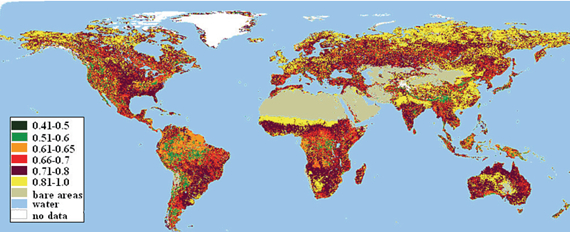• Clumping has an effect on the radiation regime inside the canopy

• $\Rightarrow$ effect on GPP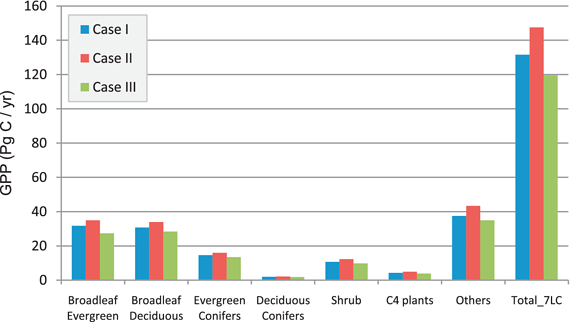• In the plot
• Case I: LAI and clumping considered
• Case II: clumping considered
• Case III: effective LAI
• Case II vs Case I: ignoring $C$ results in an overestimation of sunlit leaves $\Rightarrow$ increase in GPP
• Case III vs Case I: underestimation of shaded leaf LAI $\Rightarrow$ decrease in GPP

### Extending a turbid medium to deal with discontinuous canopies¶

• Need to deal with the mutual shadowing of e.g. tree crowns, soil, etc. $\Rightarrow$ Geometrical optics (GO)
• A first stage is to assume that crowns are turbid mediums
• Calculate scattering & attenuation
• Deal with shadowing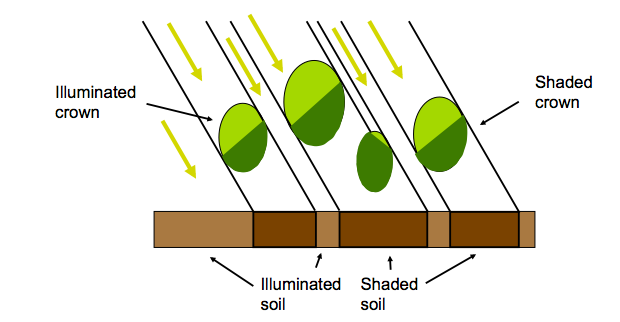### Using models to "validate" other models¶

• Hard to measure many processes (e.g. contribution of multiple scattering)
• $\Rightarrow$ use simpler models as a surrogate reality
• $\Rightarrow$ aim here is to understand e.g. effects of assumptions, etc
• RAdiative transfer Model Intercomparison (RAMI)

Summary

1. Turbid medium approximation
2. 1st O: attenuation & volume scattering
• leaf angle distribution, geometry, LAI...
• Assumptions to make modelling tractable
3. Multiple scattering
• $p$-theory
4. Hotspot effect
5. Discontinuous canopies
6. RAMI-type efforts